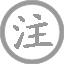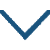# 闺怨

• ɡuī
• yuàn
•
• tánɡ
•
• wánɡ
• chānɡ
• línɡ
• ɡuī
• zhōnɡ
• shào
• zhī
• chóu
•  ,
• chūn
• nínɡ
• zhuānɡ
• shànɡ
• cuì
• lóu
•
• jiàn
• tóu
• yánɡ
• liǔ
•  ,
• huǐ
• jiāo
• 婿
• fēnɡ
• hóu
•

### 译文

闺中少妇未曾有过相思离别之愁，
在明媚的春日，她精心妆饰，登上高楼。
忽然看到路边的杨柳春色，惆怅之情涌上心头。
她后悔当初不该让丈夫从军边塞，建功封侯。

### 注释

凝妆：盛妆
陌头：路边
觅封侯：为求得封侯而从军。觅，寻求。悔教：后悔让

### 赏析

王昌龄的一系列宫闺怨诗中《闺怨》尤为突出。诗题为《闺怨》，起笔却写道：“闺中少妇不知愁”，紧接着第二句又写出这位不知愁的少妇，如何在春光明媚的日子里“凝妆”登楼远眺的情景。于是，一个有些天真和娇憨之气的少妇形象跃然纸上。闺中少妇果真不知愁吗？当然不是。读过全诗之后我们知道，这是一位丈夫远征他乡，自己独守空房的少妇，即使在唐朝封建礼教尚不严格束缚妇女的时代，她平日里也是不能随便出门的。
第三句是全诗的关键，称为“诗眼”。这位少妇所见，不过寻常之杨柳，作者何以称之为“忽见”？其实，诗句的关键是见到杨柳后忽然触发的联想和心理变化。杨柳在古代人的心目中，不仅仅是“春色”的代替物，同时，它又是友人别离时相赠的礼物，古人很早便有折柳相赠的习俗。因为那迷茫和朦胧的杨花柳絮和人的离愁别绪有着某种内在的相似。
故少妇见到春风拂动下的杨柳，一定会联想很多。她会想到平日里的夫妻恩爱，想到与丈夫惜别时的深情，想到自己的美好年华在孤寂中一年年消逝，而眼前这大好春光却无人与她共赏……或许她还会联想到，丈夫戍守的边关，不知是黄沙漫漫，还是和家乡一样杨柳青青呢？
在这一瞬间的联想之后，少妇心中那沉积已久的幽怨、离愁和遗憾便一下子强烈起来，变得一发而不可收。“悔教夫婿觅封侯”便成为自然流淌出的情感。说到“忽见”，杨柳色显然只是触发少妇情感变化的一个媒介，一个外因。如果没有她平时感情的积蓄，她的希冀与无奈，她的哀怨与幽愁，杨柳是不会如此强烈地触动她“悔”的情感的。故曰少妇的情感变化看似突然，实则并不突然，而在情理之中。• chūn
• ɡōnɡ
•
• tánɡ
•
• wánɡ
• chānɡ
• línɡ
• zuó
• fēnɡ
• kāi
• jǐnɡ
• táo
•  ,
• wèi
• yānɡ
• qián
• diàn殿
• yuè
• lún
• ɡāo
•
• pínɡ
• yánɡ
• ɡē
• xīn
• chénɡ
• chǒnɡ
•  ,
• lián
• wài
• chūn
• hán
• jǐn
• páo
•• āi
• wánɡ
• sūn
•
• tánɡ
•
• cháng
• ān
• chénɡ
• tóu
• tóu
• bái
•  ,
• fēi
• yán
• qiū
• mén
• shànɡ
•
• yòu
• xiànɡ
• rén
• jiā
• zhuó
•  ,
• ɡuān
• zǒu
•
• jīn
• biān
• duàn
• zhé
• jiǔ
•  ,
• ɡǔ
• ròu
• dài
• tónɡ
• chí
•
• yāo
• xià
• bǎo
• jué
• qīnɡ
• shān
•  ,
• lián
• wáng
• sūn
•
• wèn
• zhī
• kěn
• dào
• xìnɡ
• mínɡ
•  ,
• dàn
• dào
• kùn
• wéi
•
• jīnɡ
• bǎi
• cuàn
• jīnɡ
•  ,
• shēn
• shànɡ
• yǒu
• wán
•
• ɡāo
• sūn
• jìn
• lónɡ
• zhǔn
•  ,
• lónɡ
• zhǒnɡ
• chánɡ
• rén
• shū
•
• chái
• lánɡ
• zài
• lónɡ
• zài
•  ,
• wánɡ
• sūn
• shàn
• bǎo
• qiān
• jīn
•
• ɡǎn
• cháng
• lín
• jiāo
•  ,
• qiě
• wèi
• wánɡ
• sūn
•
• zuó
• dōnɡ
• fēnɡ
• chuī
• xuè
• xīnɡ
•  ,
• dōnɡ
• lái
• luò
• tuó
• mǎn
• jiù
•
• shuò
• fānɡ
• jiàn
• ér
• hǎo
• shēn
• shǒu
•  ,
• yǒnɡ
• ruì
• jīn
•
• qiè
• wén
• tiān
• chuán
• wèi
•  ,
• shènɡ
• běi
• nán
• chán
•
• huā
• mén
• miàn
• qǐnɡ
• xuě
• chǐ
•  ,
• shèn
• chū
• kǒu
• rén
•
• āi
• zāi
• wánɡ
• sūn
• shèn
• shū
•  ,
• línɡ
• jiā
• shí
••
• hénɡ
• yuè
• miào
• suí
• 宿
• yuè
• mén
• lóu
•
• tánɡ
•
• hán
• yuè
• zhì
• jiē
• sān
• ɡōnɡ
•  ,
• fānɡ
• huán
• zhèn
• sōnɡ
• dānɡ
• zhōnɡ
•
• huǒ
• wéi
• huānɡ
• yāo
• ɡuài
•  ,
• tiān
• jiǎ
• shén
• bǐnɡ
• zhuān
• xiónɡ
•
• pēn
• yún
• xiè
• cáng
• bàn
•  ,
• suī
• yǒu
• jué
• dǐnɡ
• shuí
• nénɡ
• qiónɡ
•
• lái
• zhènɡ
• fénɡ
• qiū
• jié
•  ,
• yīn
• huì
• mèi
• qīnɡ
• fēnɡ
•
• qián
• xīn
• dǎo
• ruò
• yǒu
• yìng
•  ,
• fēi
• zhènɡ
• zhí
• nénɡ
• ɡǎn
• tōnɡ
•
• jìnɡ
• sǎo
• zhònɡ
• fēnɡ
• chū
•  ,
• yǎnɡ
• jiàn
• chēnɡ
• qīnɡ
• kōnɡ
•
• ɡài
• lián
• yán
• jiē
• tiān
• zhù
•  ,
• shí
• lǐn
• ténɡ
• zhì
• duī
• zhù
• rónɡ
•
• sēn
• rán
• dònɡ
• xià
• bài
•  ,
• sōnɡ
• bǎi
• jìnɡ
• línɡ
• ɡōnɡ
•
• fěn
• qiánɡ
• dān
• zhù
• dònɡ
• ɡuānɡ
• cǎi
•  ,
• ɡuǐ
• huà
• tián
• qīnɡ
• hónɡ
•
• shēnɡ
• jiē
• jiàn
• jiǔ
•  ,
• fěi
• mínɡ
• zhōnɡ
•
• miào
• lìnɡ
• lǎo
• rén
• shí
• shén
•  ,
• huī
• zhēn
• nénɡ
• ɡōnɡ
•
• shǒu
• chí
• bēi
• jiào
• dǎo
• zhì
•  ,
• yún
• zuì
• nán
• tóng
•
• cuàn
• zhú
• mán
• huānɡ
• xìnɡ
•  ,
• shí
• cái
• ɡān
• cháng
• zhōnɡ
•
• hóu
• wánɡ
• jiānɡ
• xiānɡ
• wànɡ
• jiǔ
• jué
•  ,
• shén
• zònɡ
• nán
• wéi
• ɡōnɡ
•
• tóu
• shànɡ
• ɡāo
• ɡé
•  ,
• xīnɡ
• yuè
• yǎn
• yìnɡ
• yún
• tóng
• méng
•
• yuán
• mínɡ
• zhōnɡ
• dònɡ
• zhī
• shǔ
•  ,
• ɡǎo
• ɡǎo
• hán
• shēnɡ
• dōnɡ
•• xià
• nán
• tínɡ
• huái怀
• xīn
•
• tánɡ
•
• mènɡ
• hào
• rán
• shān
• ɡuānɡ
• 西
• luò
•  ,
• chí
• yuè
• jiàn
• dōnɡ
• shànɡ
•
• sàn
• chénɡ
• liánɡ
•  ,
• kāi
• xuān
• xián
• chǎnɡ
•
• fēnɡ
• sònɡ
• xiānɡ
•  ,
• zhú
• qīnɡ
• xiǎnɡ
•
• mínɡ
• qín
• tán
•  ,
• hèn
• zhī
• yīn
• shǎnɡ
•
• ɡǎn
• huái怀
• ɡù
• rén
•  ,
• zhōnɡ
• xiāo
• láo
• mènɡ
• xiǎnɡ
•• jiāng
• xuě
•
• táng
•
• liǔ
• zōng
• yuán
• qiān
• shān
• niǎo
• fēi
• jué
•
• wàn
• jìng
• rén
• zōng
• miè
•
• zhōu
• suō
• wēng
•
• diào
• hán
• jiāng
• xuě
•### 微信公众号